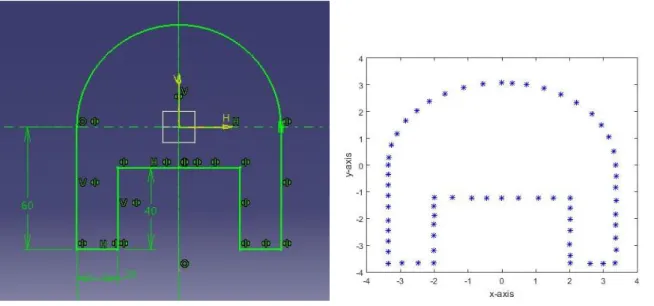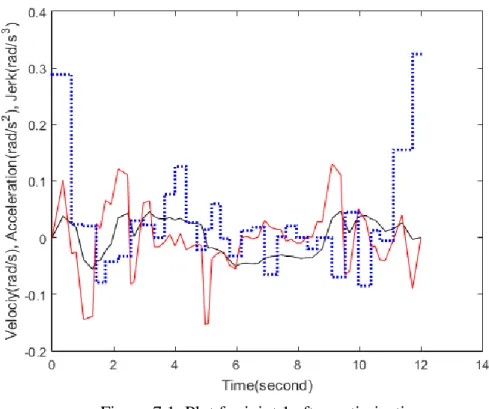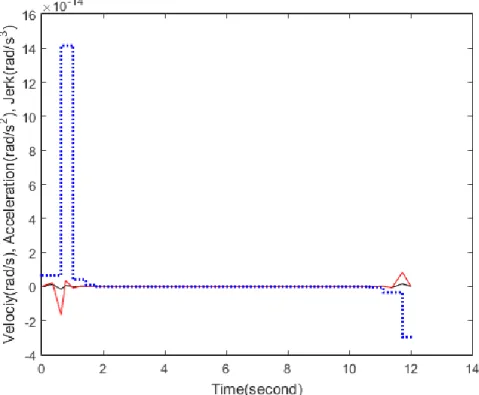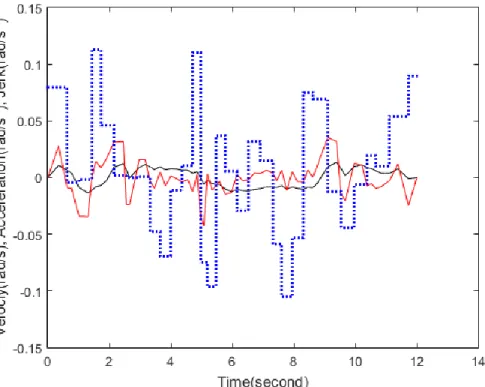# Off-Line Optimal Trajectory Planning of a 6 DOF Robot Manipulator Using

N/A
N/A
Protected

Share "Off-Line Optimal Trajectory Planning of a 6 DOF Robot Manipulator Using"

Copied!
77
0
0

Full text

We, the undersigned, having checked the above-mentioned thesis and the student's official record book(s), declare our approval of the thesis submitted in partial fulfillment of the requirements for the degree of Master of Technology in Industrial Designation National Institute of Technology Rourkela. This is to prove that the work presented in the thesis titled Off-Line Optimal Trajectory Planning of a 6 DOF Robotic Manipulator Using Multiple Objective Functions with Kinematic Constraints submitted by Amit Bhowmick, Roll Number 215ID1172, is a research record our original. supervision and guidance in partial fulfillment of the Master of Technology in Industrial Design degree requirements. Offline trajectory planning means planning the robot's motion before implementing it in the real world.

During operation, when a shock occurs, the robot deflects the end effector from its predetermined path. To reduce jerking, it is important to ensure continuity of speed and acceleration at each of the selected points.

Nomenclature

## Introduction

• Background
• Objectives
• Motivation
• Outline of the thesis

Path planning refers to the generation of the geometric path based on Cartesian space or joint space coordinates without considering the kinematic or dynamic parameters. In other words, here the main objective is to optimize the total travel time of the robot. To obtain the feasible optimal trajectory, it is also important to consider the physical limitations of the robot.

Here are the specific objectives: (i) Formation of the optimal trajectory considering various parameters responsible for producing jerks; (ii) development of the optimal algorithm; and (iii) experimentally validating that the robot's performance can be improved using the development method. In short, it can be said that motion planning of the robot is important for the performance of the robot to increase production with high accuracy.

## Literature Survey

### Off-line trajectory planning

• Total operation time minimization problem
• Energy optimization problem
• Jerk optimization problem

Optimization problems based on time minimization are important for industrial robots because they increase productivity by increasing the speed of the robot. Here, a kinematic approach is used and a complete minimization problem is derived based on the physical constraints of the robot manipulator. It was found that although the time optimization technique produces a faster path, it reduces the smoothness of the path.

Actuator torque is assumed as objective function and it is assumed that energy during movement of the robot. As stated in the previous sections, offline trajectory planning involves planning the end-effector trajectory before deploying the robot to the real world.

### On-line trajectory planning

Authors describe in  that jerk optimization trajectory planning is similar to the human arm movements. Here interval analysis based min-max optimization technique is used to solve the optimization problem. In another literature , authors described the correlation between jerk-minimized trajectory and human arm movement.

Another type of path planning is online path planning, where planning is done to track the path followed by the robot.

## Problem Description

Methodology

### Terminologies

• Robot manipulator
• Wrist of the robot
• End-effector

Like the human body, they are used as muscles to help the robot change its configuration.

Spline interpolation

## Description of the Robot

• Robot kinematics
• Estimation of joint displacements

To optimize the entire problem, it is important to define the maximum values ​​of the kinematic constraints. Using them, the origin oi of the frame Bi can be moved to the frame Bi1. It is also the distance between the xi1 and xi axes. e) i is the joint angle and it is measured along the zi1 axis.

The second approach is to first determine the Cartesian coordinates of the position of the end effector on the desired path. First the user must define the transformation matrix in terms of the position and orientation of the end effect. Before performing the inverse kinematics problem, the user must define the T60 vector in terms of orientation and end effector positions.

Since the closure of the path is required, it makes sense to define an additional point which is exactly equal to the starting point. These are the coordinates of the selected points on the predefined path as mentioned in Figure-4. Estimating the end effect positions, they are plotted in MATLAB 2015a as shown in Figure-5 to verify that the points are selected correctly.

The next task is to use inverse kinematics to obtain joint displacements at selected points. Before using inverse kinematics, it is important to define the correct transformation matrix containing the orientation. Joint movements for 64 points obtained after using inverse kinematics are given in the following table.Diagram shown in the Figure-4.3 is drawn in CATIA V5. It is expected that the robot will follow this path

## Time Intervals and Kinematic Constraints Calculation

### Time intervals calculation

As the end effector moves from point 1 to point 2, each of the six joints will simultaneously change its initial position. If hi ti1ti represents the time interval for moving from point i to point i1 on a predetermined route, there will be a total of n1 time intervals. Since the robot has a total of six joints, the time taken by the end effector to reach from point 1 to point 2 is equal to the maximum time taken by each joint to move from the initial position to the next predetermined position as indicated in table-4.5.

Where H is the set of initial time intervals, this can also be called as an upper bound.

### Kinematic constraints calculation

• Velocity constraints

Now to obtain the expression for the velocity, it is required to differentiate equation (5.12) with respect to time and the expression comes as. For example, if point 1, point 2 and point 3 are considered on the road, the time instances at these three points are t1,. To ensure continuity, point 2 of the first polynomial must coincide with point 2 of the second polynomial.

Where A is the matrix of coefficients of the matrix Q and the matrix B contains the right-hand side of the above equations. Array A contains only time intervals, while array B contains values ​​of positions, time intervals, initial and final values ​​of velocity and acceleration. Therefore, the derivative of a second-order polynomial function, or acceleration, is a linear function of time.

## Optimization Process

Optimization problem definition

### Optimization procedure

Here, Kt and Kj are the weights of the total time and total square hook functions in the objective functions. In this step, the Taylor series is applied to all KKT terms to determine the relationship between . Now the second-order partial derivative of the Lagrange function with respect to the time interval h, also known as the Hessian matrix, is given by .

Now the other four derivatives of Lagrange function with respect to hand  are given as below. Now these equations can be interpreted as the exact KKT terms of a quadratic programming problem. If an incremental or adverse change, the time interval meets a predefined tolerance value, the solution is terminated and an optimal solution is obtained.

Define KKT conditions and apply Taylor series to them, which is equivalent to a quadratic programming problem (QP).

## Simulation and Experimental Results

### Results before optimization process

The initial time responses at each of the 64 points can be calculated from the table above.

Joint 2 Joint 3 Joint 4 Joint 5 Joint 6

• Results after optimization
• Discussion
• Comparison of results

This table shows the time intervals and time responses can be calculated from this table. Following tables show the calculated values ​​of the velocities, accelerations and jerk values ​​of all joints and at all points selected on the predetermined path. In table 7.2 and table 7.6 it can be easily seen that velocities at each point for each joint are increased after optimization.

The diagrams in Figure 7.1 through Figure 7.6 show the velocity, acceleration and shock value pattern of all joints after optimization. Each of the six diagrams shows that the acceleration is a linear function of time and that the jerk remains constant for each segment of the spline curve. It was previously mentioned that the main task here is to optimize the total operating time and the total shock value.

From figure 7.7 to figure 7.12 a clear comparison can be made for jerk before optimization and after optimization.

## COMPERASION OF JERK VALUES FOR ALL JOINTS

### Experimental results

In the experiment, it is found that the experimentally obtained path differs from the theoretically obtained path. The total travel time was also around 18 seconds, which is more than the theoretically optimal time obtained.

## Conclusion and Future Scope

Future scope

P P Kumar, and B B V L Deepak; "Design and Ergonomic Evaluation of Multipurpose Student's Bed", in ICoRD'15–Research into Design through Boundaries Volume 1 (pp. 421-430). D R Parhi, B B V L Deepak, J Mohana, R Ruppa and M Nayak; "Immunized navigational controller for mobile robot navigation," in Soft Computing Techniques in Vision Science (pp. 171-182). Lecture Notes in Electrical Engineering, In Proceedings of the International Conference on Signal, Networks, Computing and Systems (pp. 315-322).

Parhi and Ravi Prakash, “Kinematic Control of a Mobile Manipulator”, International Conference on Signal, Networks, Computers and Systems, February 25 - 27, 2016, JNU, New Delhi. Kinematic modeling and simulation of manipulator for performing welding operations with arbitrary weld joint profiles, Lecture Notes in Electrical Engineering, in proceedings of the International Conference on Signals, Networks, Computers and Systems (pp. 291-300). B B V L Deepak, Alok KJ and DR Parhi; “Path planning of an autonomous mobile robot using an artificial immune system,” Proceedings of National Conference on Emerging Trends in Computing and Information Technology (NCETCIT-2011).

Kinematic control of a mobile manipulator, Lecture Notes in Electrical Engineering, in Proceedings of the International Conference on Signaling, Networks, Computing and Systems (pp. 339- 346). D R Parhi, and B B V L Deepak; "Path Generation of a Differential Mobile Robot using Particle Swarm Optimization", International Journal of Artificial Intelligence and Computational Research, Vol.4 No.1, pp. B B V L Deepak, D R Parhi, and J R Ruppa; "Immunised Navigational Controller for Mobile Robot Navigation", Proceedings of International Conference on Artificial Intelligence and Soft Computing (ICAISC 2011), Bhubaneswar, pp.

B B V L Deepak, DR Parhi and Subhasri K; “Innate immune based path planner of an autonomous mobile robot”, International Conference on Modelling, Optimization and Computing (ICMOC – 2012). E Elias, B B V L Deepak, D R Parhi and J Srinivas; "Design & Kinematic Analysis of an Articulated Robotic Manipulator", Proceedings of International Conference on Mechanical and Industrial Engineering (ICMIE-2012), Goa, pp B B V L Deepak, D R Parhi and Devidutta N.; "Development of forward and inverse kinematic models for 5-axis articulated manipulator", Proceedings of 4th International and 25th All India Manufacturing Technology, Design and Research Conference, jadavpur university, pp.102-106.

Figure+7

References

Related documents

2 hrs 2.5 Theory of organizational structures - nature and consequence of structure 2 hrs 3 Module B: Impact of structure, organization change and intervention strategy 3.1

Since it costs Rs 8 and 10 to make a biscuit packet by regular time and overtime and it costs Rs 6 and 7 respectively to make a cake by regular time and overtime, the objective function

Invasive Fungal infection in Acute Myeloid Leukemia Associated with Myeloid sarcoma of Sinonasal Cavity a rare case – Indian Journal of Otolaryngology & Head & Neck Surgery National

When we talk first step the energy is transferred from foot to upper body parts and we start moving, during the process of walking inertia keeps on changing increases when we set foot

4 RELATION BETWEEN ASTROLOGY AND MANAGERIAL SKILL Position of Mars has an impact on Courage factor in Managerial skill, Position of Mercury has an impact on Intellectuality angle,

Self empowerment means that women gain autonomy, are able to set their own agenda and are fully involved in the economic, political and social decision making process".. 3.1 Types of

43 Figure 4-8 Stress-strain curve for 15 minutes of extrusion for set-2, set-3 and set-4 ...temperature setting 4.1.6 Summary of wire testing results All the previously

List of Symbols GV, E Communication graph with the set of vertices and edges V and E respectively TuVT, ET The tree rooted at nodeu with the vertex and edge set VT,ET, respectively N

Abstract Key words : condition spectrum, vector valued analytic maps, maximum modu- lus principle, upper and lower hemicontinuous correspondence,p, q−−pseudo spectrum.. For 0 < < 1 and